# Current in the conductor

Calculate the current in the conductor (in mA) if it is connected to a 4.5 V voltage source and its resistance is 20 (ohm).

I =  225 mA

### Step-by-step explanation: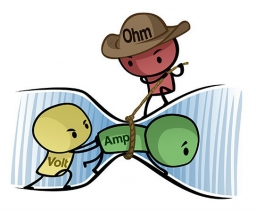Did you find an error or inaccuracy? Feel free to write us. Thank you!## Related math problems and questions:

• Mains voltageHow much electric current flows through the appliance with a resistance of 40kΩ, which is connected to the mains voltage (230 V)?
• Two resistorsTwo resistors 20 Ω and 60 Ω are connected in series and an external voltage of 400 V is connected to them. What are the electrical voltages on the respective resistors? Please comment!
• Resistance of the resistorThe resistor terminals have a voltage of 20 V and a current of 5 mA is passed through. What is the resistance of the resistor?
• Closed circuitIn a closed circuit, there is a voltage source with U1 = 12 V and with an internal resistance R1 = 0.2 Ω. The external resistance is R2 = 19.8 Ω. Determine the electric current and terminal voltage.
• Lowest voltage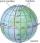Three resistors with resistors R1 = 10 kΩ, R2 = 20 kΩ, R3 = 30 kΩ are connected in series and an external voltage U = 30 V is connected to them. On which resistor is the lowest voltage?
• Effective and mean voltage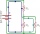A voltage divider consisting of resistors R1 = 103000 Ω and R2 = 197000 Ω is connected to the ideal sine wave voltage source, R2 is connected to a voltmeter which measures the mean voltage and has an internal resistance R3 = 200300 Ω, the measured value i
• ResistanceDetermine the resistance of the bulb with the current 200 mA and is in a regular lamp (230V).
• The bulbWhat is the resistance of a filament lamp when it is connected to a 9V battery and has a current of 120 mA?
• Direction indicatorAs that which the electrical voltage is connected to the lamp direction indicator 7400 mOhm resistance when current of 1.6 A passes through it?
• Filament of bulbThe filament of bulb has a 1 ohm resistivity and is connected to a voltage 220 V. How much electric charge will pass through the fiber when the electric current passes for 10 seconds?
• Serial connectionIf the voltage on the first resistor is 6 V and the resistance ratio of the first and second resistors is 1: 3, what will be the voltage across the second resistor if they are connected in series?
• Resistor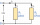Resistor 1 with a resistance of 100 Ohms and resistor 2 with a resistance of 400 Ohms are connected side by side in the circuit. There is a voltage of 80V between the resistor terminals. a) Draw a circuit diagram and write the entered quantities in it b)
• ResistanceA resistor having an electrical resistance of 1.5 k ohms passes an electrical current of 0.1 A. Calculate what voltage is between the terminals of the resistor.
• Circuit voltageCalculate the closed-circuit voltage. Calculate the power on the resistors. R1 = 30 ohms, R2 = 10 ohms. I = 0.1A. U =?. The resistors are connected in series, one after the other.
• The output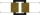The output voltage of the transformer is 880 V. The secondary coil has 1200 turns. Find the voltage to which the primary coil is connected and how many turns it has if a current of 1 A flows through it. Transformation ratio k = 4. What current flows throu
• The bulbThe bulb has a reading of 6V-0.05 A, what current flows through the bulb if we connect it to a cell with a voltage of 12V?
• Resistance - ohmsIf the resistance of the first resistor is 300 ohms and the resistance of the second resistor is 100 ohms. What is the resulting resistance?